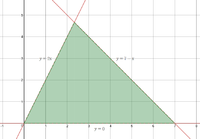# Find the point whether lies in the Cartesian plane.

#### acemi123

##### New member
In the Cartesian plane, let us consider the triangle formed by the three lines r1 : y = 0, r2 : y = 2x, r3 : y = -x + 7. Which of the following points lies inside the triangle?

(A) P=(-3;2)
(B) P=(1;-3)
(C) P=(3;3)
(D) P=(4;4)
(E) P=(3;5)

#### MarkFL

##### Super Moderator
Staff member
In order for a point $$(x,y)$$ to lie within the resulting triangle, it must simultaneously satisfy the following 3 inequalities:

$$\displaystyle 0<y$$

$$\displaystyle y<2x$$

$$\displaystyle y<7-x$$

Can you find the single given point that does?

•topsquark

#### acemi123

##### New member
In order for a point $$(x,y)$$ to lie within the resulting triangle, it must simultaneously satisfy the following 3 inequalities:

$$\displaystyle 0<y$$

$$\displaystyle y<2x$$

$$\displaystyle y<7-x$$

Can you find the single given point that does?
the answer is (3;3) Your formula is correct, can you explain how this formula works, please. Depend on which conditions? Does the order of lines matter? Is there a general rule?

•MarkFL

#### MarkFL

##### Super Moderator
Staff member
the answer is (3;3) Your formula is correct, can you explain how this formula works, please. Depend on which conditions? Does the order of lines matter? Is there a general rule?
Consider a plot of the 3 given lines, with the triangular region bounded by these lines shaded in green:Now, we see that the bottom of the triangle lies along the line $$y=0$$ and so the $$y$$-coordinates of all points within the triangle must satisfy:

$$\displaystyle 0<y$$

Next, observe that the left side of the triangle lies along the line $$y=2x$$, so all points must be to the right of that line. If we pick such a point like $$(1,1)$$, and substitute that into the line, we find:

$$\displaystyle 1<2(1)$$

And so all points within the triangle also must satisfy:

$$\displaystyle y<2x$$

Finally, we see the right side of the triangle lies along the line $$y=7-x$$ and if we again use the point $$(1,1)$$, we find:

$$\displaystyle 1<7-1$$

And so all points within the triangle also must satisfy:

$$\displaystyle y<7-x$$

And thus we may conclude that any point $$(x,y)$$ that simultaneously satisfies all 3 conditions must lie within the triangular region bounded by the 3 given lines.

•acemi123